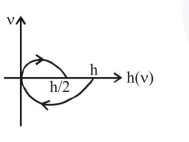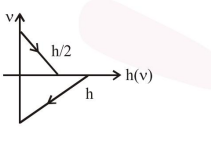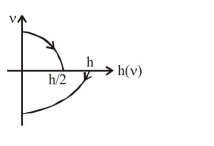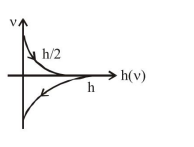# A Tennis ball is released from a height $h$ and after freely falling on a wooden floor it

Question:

A Tennis ball is released from a height $h$ and after freely falling on a wooden floor it rebounds and reaches height $\frac{\mathrm{h}}{2}$. The velocity versus height of the ball during its motion may be represented graphically by : (graph are drawn schematically and on not to scale)

1.2.3.4.Correct Option: , 3

Solution:

Velocity at ground (means zero height) is nonzero therefore one is incorrect and velocity versus height is non-linear therefore two is also incorrect.

$v^{2}=2 g h$

$\mathrm{v} \frac{\mathrm{dv}}{\mathrm{dh}}=2 \mathrm{~g}=$ const.

$\frac{\mathrm{dv}}{\mathrm{dh}}=\frac{\text { constant }}{\mathrm{v}}$

Here we can see slope is very high when velocity is low therefore at Maximum height the slope should be very large which is in option 3 and as velocity increases slope must decrease there for option 3 is correct.